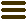The Software Design Metrics tool for the UMLChecking the Layout of UML Diagrams with SDMetrics

July 18, 2012, Jürgen Wüst. Category: Tips & Tricks

In the previous post we have discussed the problem of diagram interchange between UML modeling tools via XMI. Using the example of Altova UModel, we have shown how to customize SDMetrics to extract tool vendor specific diagram information from XMI files.

In this post, we will continue with this example and have SDMetrics automatically check a simple UML diagram layout guideline. The guideline says that super-classes on a class diagram should be located above their sub-classes (see, e.g., Scott Ambler’s UML class diagram guidelines, or this blog on UML diagram best practices). Placing sub-classes above their super-classes confuses readers and makes the diagram harder to understand (by the way, if anybody knows of any empirical work to back this hypothesis up with data, please let me know).

The strategy

In the previous post we have seen that a UML diagram consists of special “diagram elements”, which are linked to the elements they represent in the model. Some diagram elements are “nodes” (or “bubbles”) representing classes, states, activities, and so forth. Other diagram elements are “connectors” (or “lines”) such as generalizations, transitions, or control flows, connecting the nodes in the diagram.

To automatically check that super-classes are located above their sub-classes, we will use the following, simple strategy: for each diagram element that represents a generalization, we check that the diagram element at the start of the arrow is located below the diagram element at the end of the arrow.

So let’s get our hands dirty. If you want to try out the examples in this post, make sure you have added the meta-model and XMI transformation extensions from the previous post.

Comparing diagram coordinates

To check if some diagram element A is placed below another diagram element B, the top coordinate of A must be below the bottom coordinate of B. Here we hit a first problem: SDMetrics’ XMI parser only extracts strings from the XMI file. Therefore, the values of the diagramelement attributes top/left/right/bottom that we defined in our previous post are strings. Comparing those attribute values directly amounts to a lexicographical comparison. For a proper, numerical comparison, we need to convert those strings to numbers. SDMetrics does not have a “parse integer” function yet, so for the time being we’ll have to provide one ourselves. The following Java class makes method Integer.parseInt() available to us in SDMetricsML expressions.

package com.acme.mysdmetricsfunctions;
import com.sdmetrics.math.ExpressionNode;
import com.sdmetrics.metrics.SDMetricsException;
import com.sdmetrics.metrics.ScalarOperation;
import com.sdmetrics.metrics.Variables;
import com.sdmetrics.model.ModelElement;

public class IntParseFunction extends ScalarOperation {
public Object calculateValue(ModelElement me, ExpressionNode expr,
Variables vars) throws SDMetricsException {

String s=evalExpression(me, expr.getOperand(0),vars).toString();
try {
int value=Integer.parseInt(s);
return Integer.valueOf(value);
} catch(NumberFormatException ex) {
return Integer.valueOf(0);
}
}
}

We register the function in the metric definition file, and can then use it to define two metrics “y1” and “y2” for diagram elements to convert the string-valued attributes “top” and “bottom” to numbers:

<scalaroperationdefinition name="parseint"
class="com.acme.mysdmetricsfunctions.IntParseFunction" />

<metric name="y1" domain="diagramelement" internal="true">
<compoundmetric term="parseint(top)"/>
</metric>
<metric name="y2" domain="diagramelement" internal="true">
<compoundmetric term="parseint(bottom)"/>
</metric>

Defining the rule

With these definitions in place, we can now write down the rule to check the relative placement of super- and sub-classes. Actually, the following rule not only checks the placement of classes, but any type of elements with a generalization between them:

<rule name="PutSuperAbove" domain="diagramelement">
<violation condition=
"typeof(element)='generalization' and (connstart.y1 lt connend.y2)"
value="'Place super-'+typeof(connend.element)+' '+connend.element.name+' above sub-'+typeof(connstart.element)+' '+connstart.element.name" />
</rule>

The condition expression first checks whether the diagram element represents a generalization. If so, it compares the top coordinate of the sub-element at the start of the connector (connstart.y1) to the bottom coordinate of the super-element at the end of the connector (connend.y2). If the former is less than (i.e., above) the latter, the rule reports a violation.

The somewhat length value expression of the rule creates a human-readable description of the problem such as “Place super-class Foo above sub-class Bar”.

Limitations

Checking diagram layouts is not exactly a strength of SDMetrics “out of the box”. Besides the problem of extracting vendor-specific diagram information from XMI, layout checks often require special graphical algorithms in order to run efficiently. The SDMetricsML used to define custom UML metrics and rules is not a general purpose programming language, and not at all suited to implement such algorithms. You can, of course, code the algorithms in Java, plug them into SDMetrics, and call them from the SDMetricsML (like SDMetrics’ algorithm to calculate the strongly connected components of a graph). But if this is the most cost-effective way to get the job done is a different question.

However, several simple checks can be realized with the existing means. For example, testing that connector lines are orthogonal, or measuring how “cluttered” diagrams are, say, in terms of “node density” or the amount of “diagram whitespace” (area not covered by nodes), should be easy to do now.